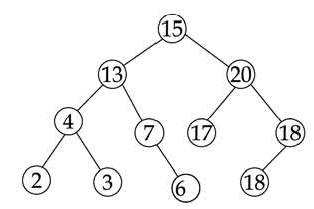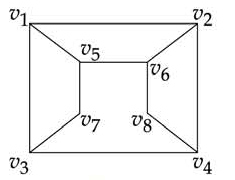# December 2015 - Paper 2

1:

How many committees of five people can be chosen from 20 men and 12 women such that each committee contains at least three women?

 A. 75240 B. 52492 C. 41800 D. 9900 Answer Report Discuss Option: B Explanation : We must choose at least 3 women, so we calculate the case of 3 women, 4 women and 5 women and by addition rule add the results. 12C3 x 20C2 + 12C4 x 20C1 + 12C5 x 20C0  = (12x11x10/3x2x1) x (20x19/2x1) + (12x11x10x9/4x3x2x1) x 20  + (12x11x10x9x8/5x4x3x2x1) x 1  = 220 x 190 + 495 x 20 + 792  = 52492 Click on Discuss to view users comments. Write your comments here:
2:

Which of the following statement(s) is/are false?

(a) A connected multigraph has an Euler Circuit if and only if each of its vertices has even degree.

(b) A connected multigraph has an Euler Path but not an Euler Circuit if and only if it has exactly two vertices of odd degree.

(c) A complete graph (Kn) has a Hamilton Circuit whenever n≥3

(d) A cycle over six vertices (C6) is not a bipartite graph but a complete graph over 3 vertices is bipartite.

 A. (a) only B. (b) and (c) C. (c) only D. (d) only Answer Report Discuss Option: D Explanation : Click on Discuss to view users comments. Write your comments here:
3:

The inorder traversal of the following tree is:A. 2 3 4 6 7 13 15 17 18 18 20 B. 20 18 18 17 15 13 7 6 4 3 2 C. 15 13 20 4 7 17 18 2 3 6 18 D. 2 4 3 13 7 6 15 17 20 18 18 Answer Report Discuss Option: D Explanation : Click on Discuss to view users comments. Write your comments here:
4:

Which of the following is/ are not true ?

(a) The set of negative integers is countable.

(b) The set of integers that are multiples of 7 is countable.

(c) The set of even integers is countable.

(d) The set of real numbers between 0 and Ih is countable.

 A. (a) and (c) B. (b) and (d) C. (b) only D. (d) only Answer Report Discuss Option: D Explanation : Click on Discuss to view users comments. Write your comments here:
5:

Consider the graph given below:The two distinct sets of vertices, which make the graph bipartite are:

 A. (v 1 , v 4 , v 6 ); (v 2 , v 3 , v 5 , v 7 , v 8 ) B. (v 1 , v 7 , v 8 ); (v 2 , v 3 , v 5 , v 6 ) C. (v 1 , v 4 , v 6 , v 7 ); (v 2 , v 3 , v 5 , v 8 ) D. (v 1 , v 4 , v 6 , v 7 , v 8 ); (v 2 , v 3 , v 5 ) Answer Report Discuss Option: C Explanation : A simple graph G=(V,E) is called bipartite if its vertex set can be partitioned into two disjoint subsets V=V 1 ⋃V 2 , such that every edge has the form e=(a,b) where aϵV 1  and bϵV 2 .   Bipartite graphs are equivalent to two-colorable graphs.   1.  Assign Red color to the source vertex (putting into set V 1 ).   2. Color all the neighbours with Black color (putting into set V 2 ).   3.Color all neighbour’s neighbour with Red color (putting into set V 1 ).   4.  This way, assign color to all vertices such that it satisfies all the constraints of m way coloring problem where m= 2.   5. While assigning colors, if we find a neighbour which is colored with same color as current vertex, then the             graph cannot be colored with 2 colors (ie., graph is not Bipartite).   So answer is option (C). Click on Discuss to view users comments. Write your comments here: